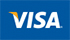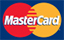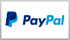# Produce

View as Grid List

Items 1-90 of 114

Page
per page
Set Descending Direction
1. apples gold
J\$201.36
2. apples red
J\$164.00
3. Apples Red Import Perry Kg Tray
J\$265.32
4. Baby Carrots per kg
J\$564.95
5. Beet Root per kg
J\$163.35
6. Black plums per kg
J\$1,565.08
7. Black Seedless Grapes per kg
J\$2,323.31
8. blueberries 170gm
J\$1,578.72
9. Bosc Pear per lb
J\$1,088.16
10. Broccoli Crowns per kg
J\$2,236.40
11. broccoli local
J\$337.16
12. cabbage (per kg)
J\$381.15
13. callaloo (per kg)
J\$204.03
14. callaloo shredded
J\$230.99
15. carrot local (per kg)
J\$355.74
16. Cauliflower Local (per kg)
J\$490.05
17. Celery Naked (per kg)
J\$278.85
18. chocolate balls
J\$114.93
19. cinnamon leaves
J\$99.00
20. coco (per kg)
J\$457.05
21. corn local
J\$417.12
22. D'Anjou Pear per lb
J\$1,757.80
23. dd herb fresh spinach
J\$445.47
24. dried peanuts (per kg)
J\$1,279.40
25. Dry Coconut per kg
J\$198.91
26. Eggplant Local per kg
J\$97.24
27. escallion (per kg)
J\$196.02
28. french lime
J\$571.99
29. fresh kale
J\$393.14
30. fruit dish (per kg)
J\$1,141.75
31. Fuji Apple per kg
J\$1,181.77
32. Gala Apples perr kgg
J\$1,344.28
33. Gala Apples KG
J\$1,113.81
34. garlic (per kg)
J\$101.81
35. geddy ss dried gungo peas 400gm
J\$132.35
36. goya black eye peas 397gm
J\$341.71
37. goya pigeon peas 397gm
J\$453.07
38. goya yellow split peas 397gm
J\$193.94
39. Granny Smith Apples per kg
J\$1,045.33
40. Grapefruit
J\$296.99
41. green banana (per kg)
J\$281.77
42. Green Bananaaaliyah per kg
J\$143.25
43. Green Seedless Grapes per kg
J\$2,163.48
44. Hot Pepper Local per kg
J\$179.74
45. Irish Potato
J\$580.42
46. Irish Potato (per kg)
J\$472.60
47. Irish Potato localll per kg
J\$386.57
48. Irish Potato per Kggg
J\$585.31
49. Irish Potatoes per kg
J\$744.84
50. JD Spice Whole Nutmeg 45g
J\$263.99
51. Jelly Coconut
J\$197.99
52. June Plum per kg
J\$329.99
53. kiwi (per kg)
J\$2,063.44
54. lemon
J\$577.47
55. Lettuce Local Per Kg
J\$334.83
56. Lettuce per kg
J\$247.72
57. lime
J\$329.99
58. local round red peas (per kg)
J\$213.29
59. local yellow squash (per kg)
J\$411.62
60. local zucchini (per kg)
J\$503.38
61. melon (per kg)
J\$376.09
62. Mustard Spinach per kg
J\$363.00
63. Okra (per kg)
J\$280.83
64. Onion perrkgg
J\$421.99
65. onion red (per kg)
J\$739.48
66. Onions per kg
J\$467.35
67. Onions per kggg
J\$283.58
68. Oranges Local Per Dozen
J\$494.98
69. Pak Choi (per kg)
J\$186.23
70. Pak Choy per kg
J\$423.94
71. peanut grain (per kg)
J\$1,828.22
72. pears bosc 80 110 19 1kg (per kg)
J\$1,518.76
73. pepper scotch bonnet
J\$99.00
74. Peppers Hot Jalapeno per kg
J\$846.12
75. phf bulgar
J\$196.17
76. picker s choice green pepper
J\$336.61
77. picker s choice red pepper
J\$336.61
78. picker s choice yellow pepper
J\$336.61
79. pimento
J\$89.09
80. pineapple (per kg)
J\$1,043.09
81. Pineapple Melon per kg
J\$326.70
82. pineapple slices dish (per kg)
J\$873.13
83. Pineapple Whole Per Kg
J\$1,045.47
84. plantain ripe (per kg)
J\$528.13
85. plummy tomato (per kg)
J\$52.25
86. pumpkin local (per kg)
J\$283.36
87. Red Apple per kg
J\$1,003.20
88. Red cabbage per kg
J\$323.84
89. Red D'Anjou Pears per kg
J\$1,246.52
90. Red Globe Grapes per kg
J\$2,316.60
View as Grid List

Items 1-90 of 114

Page
per page
Set Descending Direction

#### Payment Option

•••